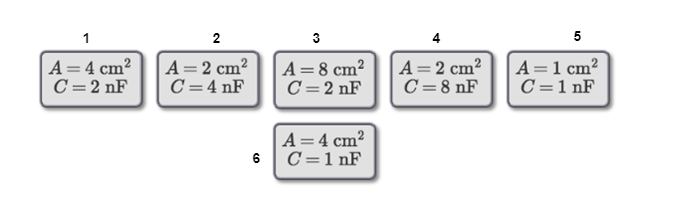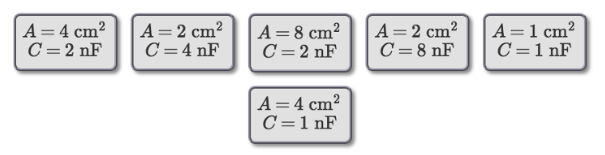# Problem: Part A. Rank the following capacitors on the basis of the dielectric constant of the material between the plates.Part B. Rank the capacitors on the basis of the charge stored on the positive plate.

###### FREE Expert Solution

Label the capacitors as follows:Part A

Capacitance us given by:

$\overline{){\mathbf{C}}{\mathbf{=}}\frac{\mathbf{A}{\mathbf{\epsilon }}_{\mathbf{0}}{\mathbf{\epsilon }}_{\mathbf{r}}}{\mathbf{d}}}$

Strength of the dielectric, εr = Cd/Aε0

91% (133 ratings)###### Problem DetailsPart A. Rank the following capacitors on the basis of the dielectric constant of the material between the plates.

Part B. Rank the capacitors on the basis of the charge stored on the positive plate.

Frequently Asked Questions

What scientific concept do you need to know in order to solve this problem?

Our tutors have indicated that to solve this problem you will need to apply the Capacitors & Capacitance concept. You can view video lessons to learn Capacitors & Capacitance. Or if you need more Capacitors & Capacitance practice, you can also practice Capacitors & Capacitance practice problems.

What professor is this problem relevant for?

Based on our data, we think this problem is relevant for Professor Wynveen's class at UMN.